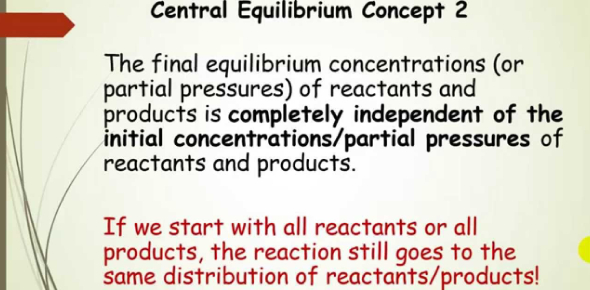# Equilibrium Concepts And Vocabulary! Trivia Quiz

9 Questions | Total Attempts: 76SettingsCan you define equilibrium concepts and vocabulary? Think you can pass this quiz? You will be asked to find the letter used for equilibrium constants, choose the correct letter in the multiple-choice questions, fill in the blank with the appropriate answer, the letter used to identify the reaction quotient. If you need to learn information on equilibrium concepts and vocabulary, this is the quiz for you.

• 1.
The letter used for equilibrium constants is
• A.

P

• B.

G

• C.

C

• D.

Q

• E.

K

• 2.
The letter use that identifies the reaction quotient
• A.

Q

• B.

R

• C.

K

• D.

P

• E.

None of the above

• 3.
The dG (read as delta G) is important as it tells you
• A.

the rate of the reaction

• B.

the change in enthalpy

• C.

the energy of activation

• D.

the spontaneity of the reaction

• E.

the equilibrium cncentration

• 4.
When the reaction quotient is equal to the equilibrium constant the concentrations of the products and reactants are at __________________ __________________
• 5.
When  the reaction quotient is greater than the equilibrium constant the reaction needs to move to the _________________to reach equilibrium
• 6.
The energy needed to get a reaction to proceed is called the
• 7.
The energy that represents the difference between products and reactants is called
• 8.
The rate determining step is also the ____________step
• 9.
When the concentration of a reactant does not affect the rate of the reaction it is a ____________order reactant
Related TopicsBack to top